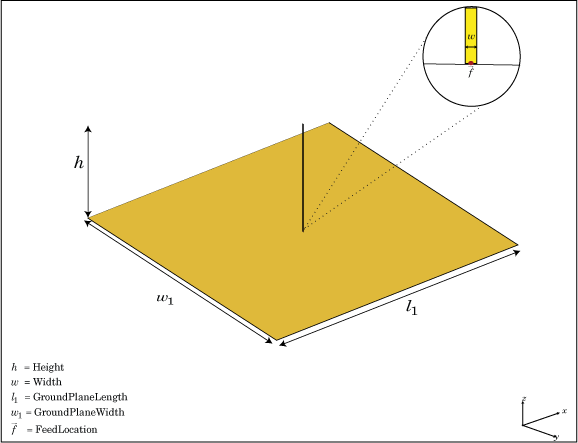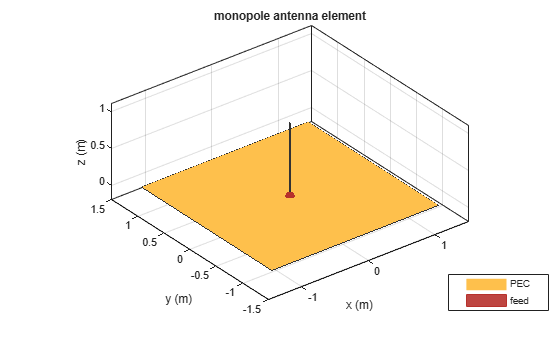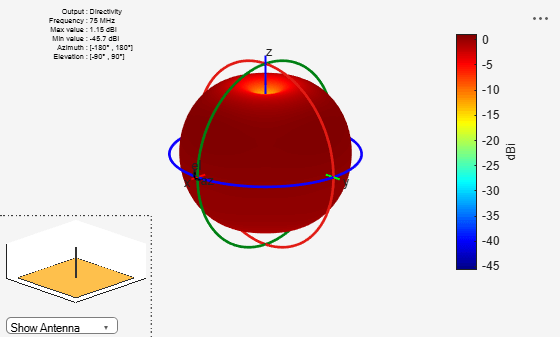# monopole

Create monopole antenna over rectangular ground plane

## Description

The `monopole` object is a monopole antenna mounted over a rectangular ground plane.

The width of the monopole is related to the diameter of an equivalent cylindrical monopole by the equation

`$w=2d=4r$`

, where:

• d is the diameter of equivalent cylindrical monopole

• r is the radius of equivalent cylindrical monopole.

For a given cylinder radius, use the `cylinder2strip` utility function to calculate the equivalent width. The default monopole is center-fed. The feed point coincides with the origin. The origin is located on the xy- plane.## Creation

### Syntax

``mpl = monopole``
``mpl = monopole(Name,Value)``

### Description

``` `mpl = monopole` creates a quarter-wavelength monopole antenna.```

example

``` `mpl = monopole(Name,Value)` creates a quarter-wavelength monopole antenna with additional properties specified by one or more name-value pair arguments. `Name` is the property name and `Value` is the corresponding value. You can specify several name-value pair arguments in any order as `Name1`, `Value1`,``` ...```, `NameN`, `ValueN`. Properties not specified retain their default values.```

## Properties

expand all

Height of vertical element along z-axis, specified as a scalar in meters. By default, the height is chosen for an operating frequency of 75 MHz.

Example: `'Height',3`

Data Types: `double`

Monopole width, specified as a scalar in meters.

Note

Monopole width should be less than `'Height'`/4 and greater than `'Height'`/1001. 

Example: `'Width',0.05`

Data Types: `double`

Ground plane length along x-axis, specified as a scalar in meters. Setting `'GroundPlaneLength'` to `Inf`, uses the infinite ground plane technique for antenna analysis.

Example: `'GroundPlaneLength',4`

Data Types: `double`

Ground plane width along y-axis, specified as a scalar in meters. Setting `'GroundPlaneWidth'` to `Inf`, uses the infinite ground plane technique for antenna analysis.

Example: `'GroundPlaneWidth',2.5`

Data Types: `double`

Signed distance from center along length and width of ground plane, specified as a two-element vector.

Example: `'FeedOffset',[2 1]`

Data Types: `double`

Type of the metal used as a conductor, specified as a metal material object. You can choose any metal from the `MetalCatalog` or specify a metal of your choice. For more information, see `metal`. For more information on metal conductor meshing, see Meshing.

Example: ```m = metal('Copper'); 'Conductor',m```

Example: ```m = metal('Copper'); ant.Conductor = m```

Lumped elements added to the antenna feed, specified as a lumped element object. For more information, see `lumpedElement`.

Example: `'Load',lumpedelement`. `lumpedelement` is the object for the load created using `lumpedElement`.

Example: ```mpl.Load = lumpedElement('Impedance',75)```

Tilt angle of the antenna, specified as a scalar or vector with each element unit in degrees. For more information, see Rotate Antennas and Arrays.

Example: `'Tilt',90`

Example: `ant.Tilt = 90`

Example: `'Tilt',[90 90]`,`'TiltAxis',[0 1 0;0 1 1]` tilts the antenna at 90 degrees about the two axes defined by the vectors.

Note

The `wireStack` antenna object only accepts the dot method to change its properties.

Data Types: `double`

Tilt axis of the antenna, specified as:

• Three-element vector of Cartesian coordinates in meters. In this case, each coordinate in the vector starts at the origin and lies along the specified points on the X-, Y-, and Z-axes.

• Two points in space, each specified as three-element vectors of Cartesian coordinates. In this case, the antenna rotates around the line joining the two points in space.

• A string input describing simple rotations around one of the principal axes, 'X', 'Y', or 'Z'.

Example: `'TiltAxis',[0 1 0]`

Example: `'TiltAxis',[0 0 0;0 1 0]`

Example: `ant.TiltAxis = 'Z'`

Note

The `wireStack` antenna object only accepts the dot method to change its properties.

Data Types: `double`

## Object Functions

 `show` Display antenna or array structure; display shape as filled patch `info` Display information about antenna or array `axialRatio` Axial ratio of antenna `beamwidth` Beamwidth of antenna `charge` Charge distribution on metal or dielectric antenna or array surface `current` Current distribution on metal or dielectric antenna or array surface `design` Design prototype antenna or arrays for resonance around specified frequency `efficiency` Radiation efficiency of antenna `EHfields` Electric and magnetic fields of antennas; Embedded electric and magnetic fields of antenna element in arrays `impedance` Input impedance of antenna; scan impedance of array `mesh` Mesh properties of metal or dielectric antenna or array structure `meshconfig` Change mesh mode of antenna structure `optimize` Optimize antenna or array using SADEA optimizer `pattern` Radiation pattern and phase of antenna or array; Embedded pattern of antenna element in array `patternAzimuth` Azimuth pattern of antenna or array `patternElevation` Elevation pattern of antenna or array `rcs` Calculate and plot radar cross section (RCS) of platform, antenna, or array `returnLoss` Return loss of antenna; scan return loss of array `sparameters` Calculate S-parameter for antenna and antenna array objects `vswr` Voltage standing wave ratio of antenna

## Examples

collapse all

Create and view a monopole of 1 m length, 0.01 m width and ground plane of dimensions 2.5mx2.5m.

`m = monopole('GroundPlaneLength',2.5,'GroundPlaneWidth',2.5)`
```m = monopole with properties: Height: 1 Width: 0.0100 GroundPlaneLength: 2.5000 GroundPlaneWidth: 2.5000 FeedOffset: [0 0] Conductor: [1x1 metal] Tilt: 0 TiltAxis: [1 0 0] Load: [1x1 lumpedElement] ```
`show(m)`Radiation pattern of a monopole at a frequency of 75 MHz.

```m = monopole('GroundPlaneLength',2.5, 'GroundPlaneWidth',2.5); pattern (m,75e6)```Balanis, C.A. Antenna Theory. Analysis and Design, 3rd Ed. New York: Wiley, 2005.

 Volakis, John. Antenna Engineering Handbook, 4th Ed. New York: Mcgraw-Hill, 2007.